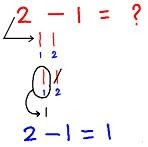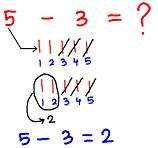# SUBTRACTION

##### Hello children, welcome back to class

SUBTOPIC: SUBTRACTION OF WHOLE NUMBERS NOT GREATER THAN 9

In subtracting 1-digit number we will subtract or minus two one-digit number and find the difference between them.

If from six things we take away 2 things, then four things are left. In arithmetic this is written in a short way as follows:

6 – 2 = 4

The symbol – is called the minus sign and shows that the number written after it is to be subtracted, or taken away, from the number written before it.

We may read 6 – 2 = 4 as ‘six minus two is equal to four’ or ‘six less two is four’.

How to subtract two one-digit numbers?

1. Here we will find 2 – 1.

Follow the steps along with the picture:1. i) 2 – 1 means start with 2 and take away 1.

(ii) From 2 strokes we take away 1 stroke and cancel the strokes.

(iii) So, 1 stroke is left.

(iv) Therefore, 2 – 1 = 1

We may read 2 – 1 = 1 as ‘two minus one is equal to one’ or ‘two less one is one’.

1. Here we will find 5 – 3.

Follow the steps of subtracting 1-digit number along with the picture:(i) 5 – 3 means start with 5 and take away 3.

(ii) From 5 strokes we take away 3 strokes and cancel the strokes.

(iii) So, 2 strokes are left.

(iv) Therefore, 5 – 3 = 2

ACTIVITY

Subtract the following digits

1. 7 – 2 =
2. 8 – 5 =
3. 5 – 3 =
4. 9 – 4 =
5. 4 – 3 =
6. 9 – 6 =
7. 4 – 1 =
8. 5 – 0 =
9. 3 – 3 =
10. 6 – 4 =
How Can We Make ClassNotesNG Better? - CLICK to Tell Us Now!